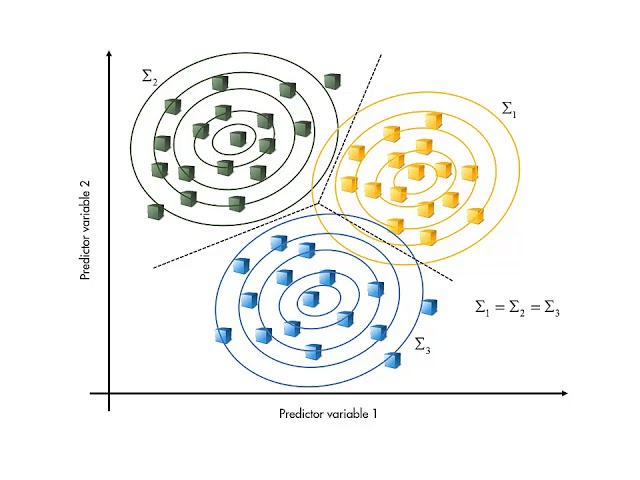# What is Discriminant analysis

##### Discriminant Analysis: An Overview

Discriminant analysis is a supervised statistical technique used to classify objects based on their characteristics or attributes. It is also known as discriminant function analysis or canonical discriminant analysis. The goal of discriminant analysis is to find the best linear combination of variables that can separate two or more groups or classes as distinctively as possible. In other words, the aim is to identify the set of variables that can best discriminate between the groups of interest. Discriminant analysis is commonly used in machine learning, pattern recognition, and data mining applications.

The basic idea behind discriminant analysis is to calculate a discriminant function that assigns a new observation to one of the known groups based on its attributes. The discriminant function is a linear combination of the predictor variables that maximizes the difference between the group means while minimizing the within-group variances. This means that the discriminant function will give more weight to the variables that are highly correlated with the group differences and less weight to the variables that do not contribute to the discrimination. Once the discriminant function is calculated, it can be used to classify new observations into the appropriate groups.

##### Types of Discriminant Analysis

There are two types of discriminant analysis: linear discriminant analysis (LDA) and quadratic discriminant analysis (QDA).

• Linear Discriminant Analysis (LDA): LDA assumes that the covariances of the predictor variables are equal across the groups. In other words, LDA assumes that the same set of predictor variables can be used to discriminate between the groups. LDA is a popular method because it is simple and efficient, especially when there are multiple groups to classify.
• Quadratic Discriminant Analysis (QDA): QDA is a more flexible method that allows for covariances that are not equal across the groups. QDA assumes that each group has its own set of predictor variables that can be used to discriminate between the groups. This means that the decision boundary between the groups is curved, rather than linear. QDA is useful when there are only a few groups to classify or when the covariance structures of the groups are different.
##### Applications of Discriminant Analysis

Discriminant analysis has many applications in various fields. Some of the common applications of discriminant analysis are:

• Medical diagnosis: Discriminant analysis can be used to diagnose diseases by classifying patients into disease or non-disease groups based on their symptoms or test results.
• Quality control: Discriminant analysis can be used to ensure that products meet certain quality standards by classifying them into acceptable or unacceptable groups based on their attributes.
• Criminal profiling: Discriminant analysis can be used to identify the characteristics of criminals based on their crime patterns or other attributes.
• Marketing: Discriminant analysis can be used to identify the characteristics of customers who are more likely to buy a certain product.
• Ecology: Discriminant analysis can be used to identify the habitats of different species based on their environmental characteristics.
• Education: Discriminant analysis can be used to predict which students are more likely to succeed in a certain program based on their attributes.

Some of the advantages of discriminant analysis are:

• Discriminant analysis is a powerful statistical tool that can identify the most important variables that discriminate between the groups.
• Discriminant analysis can handle data that are continuous, categorical, or a mixture of both.
• Discriminant analysis can be used to classify objects into two or more groups, depending on the application.
• Discriminant analysis is a computationally efficient method that can handle large datasets.

Some of the disadvantages of discriminant analysis are:

• Discriminant analysis assumes that the data are normally distributed and that the covariances of the predictor variables are equal across the groups.
• Discriminant analysis may not work well if there are too many predictor variables relative to the number of observations, or if the predictor variables are highly correlated with each other.
• Discriminant analysis requires a priori knowledge of the groups and their characteristics.
• Discriminant analysis is a linear classification method that may not work well if the decision boundary between the groups is curvilinear.
##### Conclusion

Discriminant analysis is a powerful statistical tool that can be used to classify objects into two or more groups based on their attributes. Discriminant analysis is a supervised learning technique that relies on a priori knowledge of the groups and their characteristics. There are two main types of discriminant analysis: linear discriminant analysis (LDA) and quadratic discriminant analysis (QDA). LDA assumes that the covariances of the predictor variables are equal across the groups, while QDA allows for unequal covariances. Discriminant analysis has many applications in various fields, including medical diagnosis, quality control, criminal profiling, marketing, ecology, and education. While discriminant analysis has some limitations, it is a useful technique that can help researchers and practitioners make informed decisions based on their data.